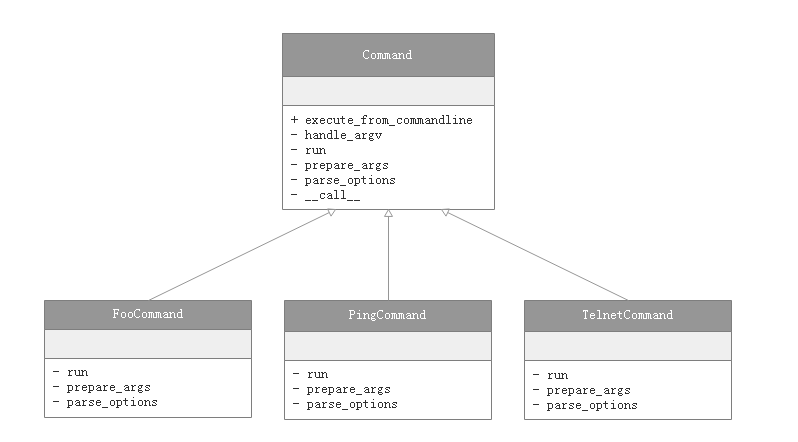### 文件组织结构

``foo/``├── foo/``│   ├── classmodule.py``│   ├── funcmodule.py``│   ├── __init__.py``│   └── __main__.py``├── LICENSE``├── README.md``└── setup.py``
1. 首先是一个大的目录，表示一个这是一个项目，应该有常写 python 代码的都比较熟悉
2. 然后是真正的应用目录，里面就存放真正的应用代码
4. setup.py 就是安装和卸载应用使用的啦

#### main 文件

``#!/usr/bin/env python``# encoding: utf-8``import sys``from .classmodule import FooCommand``def main():``    argv = sys.argv``    cmd = FooCommand()``    cmd.execute_from_commandline(argv)``if __name__ == '__main__':``    main()``

``foo ping www.google.com``foo telnet www.google.com``• execute_from_commandline：这个方法是这个类的入口，调用从此开始
• handle_argv：根据命令行的参数设置我们的应用程序
• prepare_args：解析必须的参数
• parse_options：解析可选参数
• __call__ : 处理执行结果
• run：真正得执行逻辑代码

#### classmodule.py

``#!/usr/bin/env python``# encoding: utf-8``from __future__ import absolute_import, print_function, unicode_literals``import os``import random``import sys``class Command(object):``    args = ''``    supports_args = True``    option_list = ()``    doc = None``    respects_app_option = True``    prog_name = 'foo'``    def __init__(self):``        pass``    def run(self, *args, **options):``        raise NotImplementedError('subclass responsibility')``    def __call__(self, *args, **kwargs):``        random.seed()  # maybe we were forked.``        self.verify_args(args)``        try:``            ret = self.run(*args, **kwargs)``            return ret if ret is not None else 'OK'``        except self.UsageError as exc:``            self.on_usage_error(exc)``            return exc.status``        except self.Error as exc:``            self.on_error(exc)``            return exc.status``    def verify_args(self, given, _index=0):``        pass``    def execute_from_commandline(self, argv=None):``        if argv is None:``            argv = list(sys.argv)``        self.prog_name = os.path.basename(argv)``        return self.handle_argv(self.prog_name, argv[1:])``    def run_from_argv(self, prog_name, argv=None, command=None):``        return self.handle_argv(prog_name,``                                sys.argv if argv is None else argv, command)``    def usage(self, command):``        return '%prog {0} [options] {self.args}'.format(command, self=self)``    def get_options(self):``        return self.option_list``    def handle_argv(self, prog_name, argv, command=None):``        options, args = self.prepare_args(``            *self.parse_options(prog_name, argv, command))``        return self(*args, **options)``    def prepare_args(self, options, args):``        pass``    def check_args(self, args):``        pass``    def error(self, s):``        pass``    def die(self, msg, status='EX_FAILURE'):``        self.error(msg)``        sys.exit(status)``    def parse_options(self, prog_name, arguments, command=None):``        self.parser = self.create_parser(prog_name, command)``        return self.parser.parse_args(arguments)``    def create_parser(self, prog_name, command=None):``        pass``    def prepare_parser(self, parser):``        pass``    def parse_preload_options(self, args):``        pass``    def preparse_options(self, args, options):``        pass``    @property``    def colored(self):``        if self._colored is None:``            self._colored = term.colored(enabled=not self.no_color)``        return self._colored``    @colored.setter``    def colored(self, obj):``        self._colored = obj``    @property``    def no_color(self):``        return self._no_color``    @no_color.setter``    def no_color(self, value):``        self._no_color = value``        if self._colored is not None:``            self._colored.enabled = not self._no_color``

#### setup.py

``from setuptools import setup``setup(``    name = 'foo',``    version = '0.1.0',``    packages = ['foo'],``    entry_points = {``        'console_scripts': [``            'foo = foo.__main__:main'``        ]``    })``